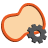Back to Adaptive Vision Studio website

You are here: Start » Filter Reference » Region Features

# Region Features

Select a filter from the list below.

Icon Name DescriptionRegionArea Computes the number of pixels contained in a region.RegionBoundingBox Computes the smallest box containing a region.RegionBoundingBox_OrNil Computes the smallest box containing a region; returns NIL if the region is empty.RegionBoundingCircle Computes the smallest circle enclosing a region.RegionBoundingCircle_OrNil Computes the smallest circle enclosing a region; returns NIL if the region is empty.RegionBoundingParallelogram Computes the smallest parallelogram containing a region.RegionBoundingRectangle Computes the smallest rectangle containing a region.RegionBoundingRectangle_FixedAngle Computes the smallest rectangle with the given orientation angle containing a region.RegionBoundingRectangle_FixedAngle_OrNil Computes the smallest rectangle with the given orientation angle containing a region; returns NIL if the region is empty.RegionBoundingRectangle_OrNil Computes the smallest rectangle containing a region; returns NIL if the region is empty.RegionCaliperDiameter Computes the longest and the shortest width of the input region measured as distance between parallel lines containing the whole region.RegionCircularity Computes the area of a region divided by the area of a circular region having the same feature.RegionContours Computes an array of closed paths corresponding to the contours of the input region.RegionConvexity Computes the area of a region divided by area of its convex hull.RegionDiameter Computes the longest segment connecting two pixels contained in region and its length.RegionEllipticAxes Computes axes of an ellipse having the same first and second order moments as the given region.RegionElongation Computes the elongation factor of a region ( perfect circle has minimal elongation equal 1.0 ).RegionHoles Creates regions of the holes of the input region.RegionInscribedBox Computes the largest box contained in a region.RegionInscribedCircle Computes the largest circle contained in a region.RegionMassCenter Computes a point with coordinates equal to the average coordinates of the region's pixels.RegionMassCenter_OrNil Computes a point with coordinates equal to the average coordinates of the region's pixels; returns NIL if the region is empty.RegionMedialAxis Computes an array of paths corresponding to the skeleton of the input region.RegionMoment Computes selected second-order moment of a region in regular and normalized ( divided by region area ) variant.RegionNumberOfHoles Computes the number of holes in a region.RegionOrientation Computes the orientation of a region as an angle of value in range 0.0 - 180.0.RegionPerimeterLength Computes the length of the input region perimeter.RegionPointRunLengths Returns the lengths of horizontal sequences of locations that constitute the input region.RegionProjection Computes the profile of the region pixel count in consecutive rows or columns.RegionRectangularity Computes the area of a region divided by the area of its bounding rectangle.